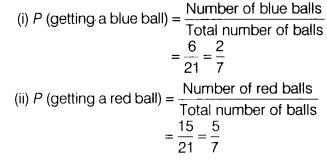# Probability of getting a blue ball is 2/3 from a bag containing 6 blue and 3 red balls

Probability of getting a blue ball is 2/3 from a bag containing 6 blue and 3 red balls. 12 red balls are added in the bag, then find the probability of getting.
(i)a blue ball. (ii) a red ball.

Given, number of blue balls in bag = 6 and the number of red balls in bag = 3 Total balls in a bag = 6+3 = 9 After adding 12 red balls,
Total number of balls = 9 +12 = 21
Number of red balls = 3+12 = 15